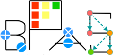Basic Polynomial Algebra Subprograms (BPAS)  v. 1.652
BPASRecursivelyViewedPolynomial< Ring, Derived > Class Template Referenceabstract

An abstract class defining the interface of a multivariate polynomial that can be viewed recursively. More...

`#include <polynomial.h>`

Simplified semantic inheritance diagram for BPASRecursivelyViewedPolynomial< Ring, Derived >:Full inheritance diagram for BPASRecursivelyViewedPolynomial< Ring, Derived >:
[legend]

## Public Member Functions

virtual Derived initial () const =0

virtual Symbol mainVariable () const =0

virtual int mainDegree () const =0

virtual Derived rank () const =0

virtual Derived tail () const =0

virtual Derived head () const =0

virtual Derived separant () const =0Public Member Functions inherited from BPASMultivariatePolynomial< Ring, Derived >
virtual void differentiate (const Symbol &)=0

virtual void differentiate (const Symbol &, int)=0

virtual Derived derivative (const Symbol &) const =0

virtual Derived derivative (const Symbol &, int) const =0

virtual Derived evaluate (int, const Symbol *, const Ring *) const =0

virtual Derived evaluate (const std::vector< Symbol > &, const std::vector< Ring > &) const =0

virtual int numberOfVariables () const =0

virtual int numberOfRingVariables () const =0

virtual Integer degree (const Symbol &v) const =0

virtual Ring coefficient (int, const int *) const =0

virtual Ring coefficient (const std::vector< int > &v) const =0

virtual void setCoefficient (int, const int *, const Ring &r)=0

virtual void setCoefficient (const std::vector< int > &v, const Ring &r)=0

virtual void setRingVariables (const std::vector< Symbol > &xs)=0

virtual std::vector< SymbolringVariables () const =0

virtual std::vector< Symbolvariables () const =0

## Detailed Description

### template<class Ring, class Derived> class BPASRecursivelyViewedPolynomial< Ring, Derived >

An abstract class defining the interface of a multivariate polynomial that can be viewed recursively.

That is, it can be viewed as a univariate polynomial with multivariate polynomial coefficients.

The documentation for this class was generated from the following file: